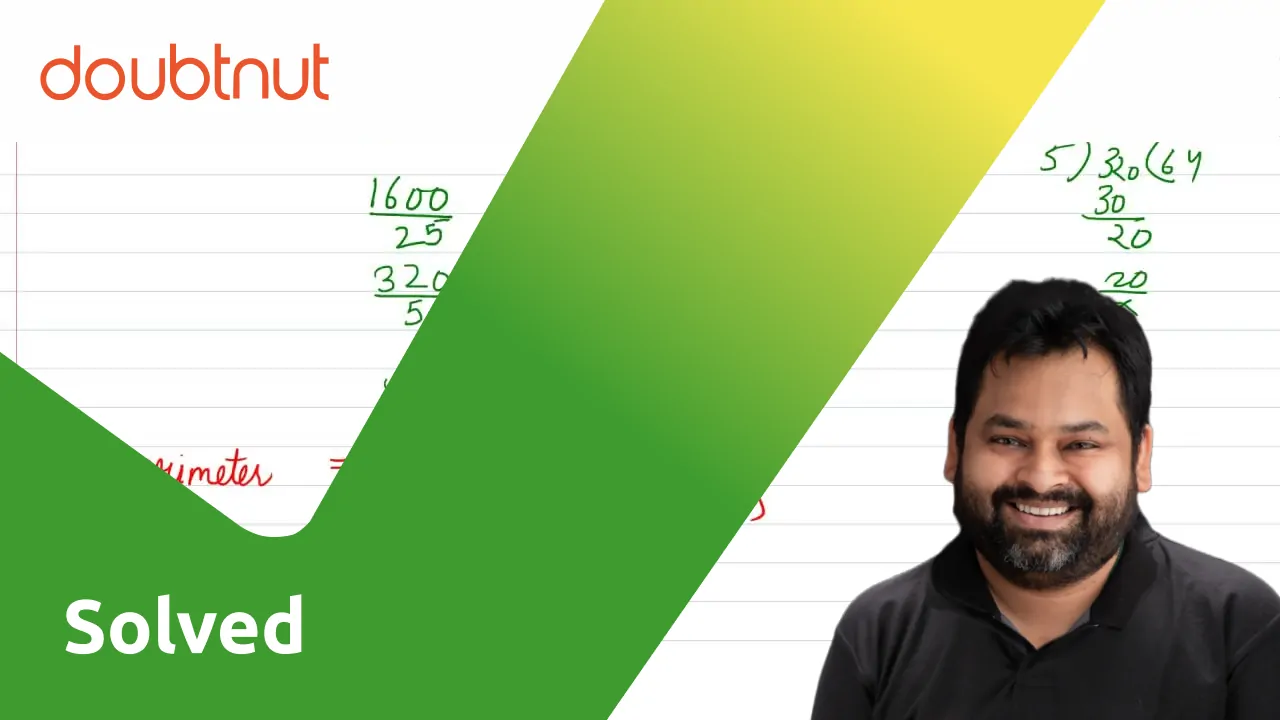Doubtnut App - Get 50 Lakh+ video solutions better than GoogleInstall Now

# The area of a square and a rectangle are equal. If the side of the square is40 cm and the breadth of the rectangle is 25 cm, find the length of therectangle. Also, find the perimeter of the rectangle.

Text Solution
Verified by Experts

## Given, side of the square = 40 cm So, area of the square = S^2 = 40×40 = 1600cm2 Given, breadth of the rectangle = 25 cm So, area of the rectangle = length × breadth = length × 25 cm Given, area of the square = area of the rectangle ⇒ 1600cm2 = length × 25 cm ⇒ length = 1600/25 = 64 cm. Therefore, length of the rectangle is 64 cm